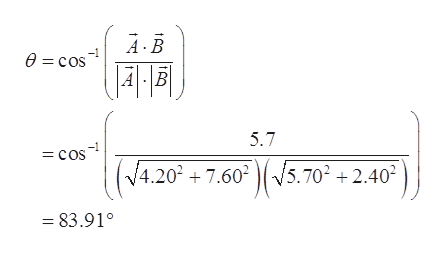# Given two vectors A⃗ = 4.20 i^+ 7.60 j^ and B⃗ = 5.70 i^− 2.40 j^ , find the scalar product of the two vectors A⃗  and B⃗ . Find the angle between these two vectors.

Question

Given two vectors A⃗ = 4.20 i^+ 7.60 j^ and B⃗ = 5.70 i^− 2.40 j^ , find the scalar product of the two vectors A⃗  and B⃗ .

Find the angle between these two vectors.

check_circle

Step 1

The scaler product of unit vectors is:

Step 2

The scalar product of the two vectors is:

Step 3

The formula for the angle be...help_outlineImage TranscriptioncloseAB 0 cos | 4 B 5.7 = cos (4.20+ 7.60 (/5.70 +2 V5.7022.402 83.91° fullscreen

### Want to see the full answer?

See Solution

#### Want to see this answer and more?

Solutions are written by subject experts who are available 24/7. Questions are typically answered within 1 hour.*

See Solution
*Response times may vary by subject and question.
Tagged in

### Vectors and Scalars